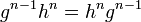# Nth power map is surjective endomorphism implies (n-1)th power map is endomorphism taking values in the center

## Statement

The following statements are equivalent for a group$G$ and an integer$n$. Suppose the$n^{th}$ power map$g \mapsto g^n$ is a surjective endomorphism (such as an automorphism) of$G$.

Then, the$(n-1)^{th}$ power map$g \mapsto g^{n-1}$ is an endomorphism of$G$ and$g^{n-1}$ is in the center of$G$ for all$g \in G$.

## Related facts

### Converse

A precise converse does not hold, but the following does: (n-1)th power map is endomorphism taking values in the center implies nth power map is endomorphism. We cannot guarantee surjectivity in general.

## Facts used

1. nth power map is endomorphism implies every nth power and (n-1)th power commute

## Proof

This proof uses a tabular format for presentation. Provide feedback on tabular proof formats in a survey (opens in new window/tab) | Learn more about tabular proof formats|View all pages on facts with proofs in tabular format

Given: A group$G$ and an integer$n$ such that$\sigma = g \mapsto g^n$ is a surjective endomorphism of$G$.

To prove:$\tau = g \mapsto g^{n-1}$ is an endomorphism of$G$ and$g^{n-1}x = xg^{n-1}$ for all$g,x \in G$.

Proof

Step no. Assertion/construction Facts used Given data used Previous steps used Explanation
1$g^{n-1}h^n = h^ng^{n-1}$ for all$g,h \in G$. Fact (1)$n^{th}$ power map is endomorphism Given+Fact direct
2$g^{n-1}x = xg^{n-1}$ for all$g,x \in G$$n^{th}$ power map is surjective Step (1) [SHOW MORE]
3 For any$g,x \in G$,$(gx)^{n-1} = g^{n-1}x^{n-1}$, so the$(n-1)^{th}$ power map is an endomorphism$n^{th}$ power map is endomorphism Step (2) [SHOW MORE]

Steps (2) and (3) complete the proof.